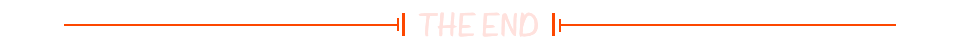-- 错误示例

``````SELECT d.dept_name,
t.avg_salary
FROM department d
JOIN (SELECT avg(e.salary) AS avg_salary
FROM employee e
WHERE e.dept_id = d.dept_id) t;
SQL 错误 : ERROR: syntax error at end of input
位置：183
``````

``````SELECT dept_id, dept_name
FROM department;
``````

``````for dept_id, dept_name in department
loop
print dept_id, dept_name
end loop
``````

SELECT 就像一个循环语句，而 LATERAL 就像是一个嵌套循环语句，对于左侧表中的每行记录执行一次子查询操作。例如，通过增加 LATERAL 关键字，我们可以修改第一个示例：

``````SELECT d.dept_name,
t.avg_salary
FROM department d
CROSS JOIN LATERAL
(SELECT avg(e.salary) AS avg_salary
FROM employee e
WHERE e.dept_id = d.dept_id) t;

dept_name  |avg_salary            |
-----------+----------------------+

``````

CROSS JOIN LATERAL 右侧的查询可以引用左侧表中的字段，以上语句为 JOIN 左侧的每个部门返回了月薪总和。

LATERAL 可以帮助我们实现一些有用的分析功能，例如以下查询返回了每个部门月薪最高的 3 名员工：

``````SELECT d.dept_name, t.emp_name, t.salary
FROM department d
LEFT JOIN LATERAL
(SELECT emp_name, salary
FROM employee e
WHERE e.dept_id = d.dept_id
ORDER BY salary DESC
LIMIT 3) t
ON TRUE;

dept_name  |emp_name|salary  |
-----------+--------+--------+

``````

``````for d in department
loop
for e in employee order by salary desc
loop
cnt++
if cnt <= 3
then
return e
else
goto next d
end
end loop
end loop
``````

``````EXPLAIN
SELECT d.dept_name, t.emp_name, t.salary
FROM department d
LEFT JOIN LATERAL
(SELECT emp_name, salary
FROM employee e
WHERE e.dept_id = d.dept_id
ORDER BY salary DESC
LIMIT 3) t
ON TRUE;

QUERY PLAN                                                                                       |
-------------------------------------------------------------------------------------------------+
Nested Loop Left Join  (cost=8.17..4439.35 rows=540 width=250)                                   |
->  Seq Scan on department d  (cost=0.00..15.40 rows=540 width=122)                            |
->  Limit  (cost=8.17..8.17 rows=1 width=132)                                                  |
->  Sort  (cost=8.17..8.17 rows=1 width=132)                                             |
Sort Key: e.salary DESC                                                            |
->  Index Scan using idx_emp_dept on employee e  (cost=0.14..8.16 rows=1 width=132)|
Index Cond: (dept_id = d.dept_id)                                            |
``````

Nested Loop Left Join 说明 PostgreSQL 使用的就是嵌套循环算法。``````

☞贾跃亭回应是否有回国打算：那必须的；顺丰将拿出 2 亿元给快递员加薪；OpenAI 宣布解散机器人团队|极客头条☞“为了拿下 Offer，我在技术面试时迎合面试官，给了错误答案！”☞Windows、Linux 纷纷被爆漏洞，黑客可直取 root 权限！``````20年前，《新程序员》创刊时，我们的心愿是全面关注程序员成长，中国将拥有新一代世界级的程序员。20年后的今天，我们有了新的使命：助力中国IT技术人成长，成就一亿技术人！